• 齐次变换矩阵
2022-04-20 14:22:58

本系列教程作者：小鱼
公众号：鱼香ROS
QQ交流群：139707339
教学视频地址：小鱼的B站
完整文档地址：鱼香ROS官网
版权声明：如非允许禁止转载与商业用途。# 7.3.1 齐次坐标变换

前面几节中，小鱼带你一起学习了使用TF进行坐标的变换，也带你通过旋转和平移求解了下坐标的变换关系，但计算的过程中旋转和平移是分开计算的，那有没有一种方法，可以让旋转矩阵和平移向量合并到同一个矩阵里呢？

答案是有的，我们可以将 3 ∗ 3 3*3 的旋转矩阵和 3 ∗ 1 3*1 的平移矩阵进行组合，并添加一行(0,0,0,1)使其变成一个 4 ∗ 4 4*4 的方阵，其组合方式如下：

有旋转矩阵
R = [ r 11 r 12 r 13 r 21 r 22 r 23 r 31 r 32 r 33 ] (旋转矩阵) R = \begin{bmatrix}{r_{11}}&{r_{12}}&{r_{13}}\\{r_{21}}&{r_{22}}&{r_{23}}\\{r_{31}}&{r_{32}}&{r_{33}}\\\end{bmatrix} \tag{旋转矩阵}
平移矩阵
P = [ x y z ] (平移矩阵) P= \begin{bmatrix}{x}\\{y}\\{z}\\\end{bmatrix} \tag{平移矩阵}

合并成齐次变换矩阵
T = [ r 11 r 12 r 13 x r 21 r 22 r 23 y r 31 r 32 r 33 z 0 0 0 1 ] (齐次矩阵) T = \begin{bmatrix}{r_{11}}&{r_{12}}&{r_{13}}&{x} \\{r_{21}}&{r_{22}}&{r_{23}}&{y} \\{r_{31}}&{r_{32}}&{r_{33}}&{z} \\0&0&0&1 \\\end{bmatrix} \tag{齐次矩阵}

为什么要这样写，我们可以简单的推导一下，矩阵是支持分块运算的，我们将上面的矩阵进行分块
T = [ R P 0 1 ] (齐次矩阵) T = \begin{bmatrix}{R}&{P} \\0&1\\\end{bmatrix} \tag{齐次矩阵}
假设 B A T ^A_BT 表示A坐标系到B坐标的齐次变换，B坐标系下的点C坐标为 C B P ^B_CP ,求C在A坐标系下的坐标 C A P ^A_CP

我们将 B A T ^A_BT C B P ^B_CP 上，可得
C A P = [ B A R B A P 0 1 ] [ C B P 1 ] = B A R C B P + B A P ^A_CP= \begin{bmatrix}{^A_BR}&{^A_BP}\\0&1\\\end{bmatrix} \begin{bmatrix}{^B_CP}\\1\\\end{bmatrix} = {^A_BR}{^B_CP}+^A_BP
根据前面学习的平移+旋转复合坐标变换公式,正确的结果如下
C A P = B A R C B P + B A P ^A_CP = {^A_BR}{^B_CP}+^A_BP
你会发现，两者最终结果完全相同，也就是说，我们的平移加旋转复合变换，可以直接用齐次变换矩阵代替。

## 1.齐次变换矩阵特性

接着我们来探索一下齐次变换矩阵的一些特性

### 2.1.齐次变换矩阵的符号表示

一般使用H或者T来表示齐次变换矩阵，矩阵的左上角标明参考坐标系，矩阵左下角标定目标坐标系，比如 B A T ^A_BT 表示A坐标系到B坐标系的变换关系(平移+旋转)

### 2.2.齐次变换矩阵的逆的几何含义

就像矩阵的逆一样，齐次变换矩阵也有逆，其的逆也有对应的几何含义，比如

比如 B A T ^A_BT 表示A坐标系到B坐标系的变换关系

那么

B A T ^A_BT 的逆 B A T − 1 = A B T ^A_BT^{-1}=^B_AT 表示B坐标系到A坐标系的变换关系

### 2.3.齐次变换矩阵的乘法的几何含义

#### 3.3.1齐次矩阵与平移向量相乘

齐次矩阵与平移向量相乘，即可求出某个向量在另一坐标系下的表示，上面例子中即是如此。

#### 3.3.2齐次矩阵与齐次矩阵相乘

齐次矩阵与齐次矩阵相乘，可以转换不同坐标系之间的关系，比如：
B A T C B T = C A T ^A_BT^B_CT=^A_CT
比如当我们有一个六自由度的机械臂，知道每两个相邻关节之间的关系，那么就可以通过其次矩阵相乘的方法求出，关节6在关节0下的位置和姿态：
1 0 T 2 1 T 3 2 T 4 3 T 5 4 T 6 5 T = 6 0 T ^0_1T^1_2T^2_3T^3_4T^4_5T^5_6T=^0_6T

## 3.练习

练习小鱼放到了下一节了，毕竟不希望大家用手来算~

技术交流&&问题求助：

• 微信公众号及交流群：鱼香ROS

• 小鱼微信：AiIotRobot

• QQ交流群：139707339

• 版权保护：已加入“维权骑士”（rightknights.com）的版权保护计划

### 作者介绍：

我是小鱼，机器人领域资深玩家，现深圳某独脚兽机器人算法工程师一枚
初中学习编程，高中开始接触机器人，大学期间打机器人相关比赛实现月入2W+（比赛奖金）
目前在输出机器人学习指南、论文注解、工作经验，欢迎大家关注小鱼，一起交流技术，学习机器人机器人学
更多相关内容
• 齐次变换矩阵 左乘 原齐次变换矩阵

clc;
clear;
close all;

% rng(0);
% rand(1)*2*pi

%% W: world coordinate system
% 尺寸4X4，齐次矩阵，齐次坐标系
W = eye(4);
W(1:3,1:3)=W(1:3,1:3)*200; % 乘以200是为了使得坐标轴变长，便于作图时候显示

%% 变换（零）
% 动态参考坐标系DRF，初始状态和世界坐标系完全重合
%（1）绕W的X轴旋转，（2）绕W的Y轴旋转，（3）绕W的Z轴旋转，
%（4）相对于W的坐标原点，沿着W的XYZ方向平移
roll_WtoDRF00 = pi/2;
pitch_WtoDRF00 = 0;
yaw_WtoDRF00 = 0;
EA_zyx_WtoDRF00 = [yaw_WtoDRF00 pitch_WtoDRF00 roll_WtoDRF00];
Tl_WtoDRF00 = [200,200,-1000]';
HTfM_WtoDRF00= genHTfM_useEularAngle(EA_zyx_WtoDRF00,Tl_WtoDRF00);
DRF00 = HTfM_WtoDRF00*W;

%% 变换（一）
% 动态参考坐标系DRF00，由变换（一）得到
% (0) DRF00平移回到和W完全重合。只有平移，不旋转
%（1）绕W的X轴旋转，（2）绕W的Y轴旋转，（3）绕W的Z轴旋转，
% (4) 把DRF00平移到，变换（一）结束时候的位置，得到DRF01。只有平移，不旋转
% 效果等价于，DRF00固定自己的坐标原点，沿着W的XYZ轴旋转
roll_DRF00toDRF01 = pi/2;
pitch_DRF00toDRF01 = 0;
yaw_DRF00toDRF01 = 0;
EA_zyx_DRF00toDRF01 = [yaw_DRF00toDRF01 pitch_DRF00toDRF01 roll_DRF00toDRF01];
R_DRF00toDRF01 = eul2rotm(EA_zyx_DRF00toDRF01,'ZYX');
HTfM_WtoDRF01 = HTfM_WtoDRF00;
HTfM_WtoDRF01(1:3,1:3) = R_DRF00toDRF01*HTfM_WtoDRF01(1:3,1:3);
DRF01 = HTfM_WtoDRF01*W;

% 画图
axis_limit = 1000;
axis_limitA = [-axis_limit,axis_limit,-axis_limit,axis_limit,-1500,300];

figure(1)
trplot(W,'frame',  'RW','color','r',  'axis',axis_limitA,  'text_opts',{'FontSize', 10, 'FontWeight', 'light'},  'view','auto',  'thick',1,  'dispar',0.8);
hold on;
plot3(W(1,4),W(2,4),W(3,4),'ro')
hold on;
trplot(DRF00,'frame',  'R00','color','g',  'axis',axis_limitA,  'text_opts',{'FontSize', 10, 'FontWeight', 'light'},  'view','auto',  'thick',1,  'dispar',0.8);
hold on;
plot3(DRF00(1,4),DRF00(2,4),DRF00(3,4),'go')
hold on;
trplot(DRF01,'frame',  'R01','color','b',  'axis',axis_limitA,  'text_opts',{'FontSize', 10, 'FontWeight', 'light'},  'view','auto',  'thick',1,  'dispar',0.8);
hold on;
plot3(DRF01(1,4),DRF01(2,4),DRF01(3,4),'bo')

%% 变换（二）
% 动态参考坐标系DRF00，由变换（一）得到。对DRF00做一下操作，得到DRF02
%（1）绕W的X轴旋转，（2）绕W的Y轴旋转，（3）绕W的Z轴旋转
% DRF00在旋转的过程中，其坐标原点在世界坐标系下的位置也会随之旋转。
roll_DRF00toDRF02 = pi/10;
pitch_DRF00toDRF02 = 0;
yaw_DRF00toDRF02 = 0;
EA_zyx_DRF00toDRF02 = [yaw_DRF00toDRF02 pitch_DRF00toDRF02 roll_DRF00toDRF02];
Tl_DRF00toDRF02 = [0,0,0]';
HTfM_DRF00toDRF02= genHTfM_useEularAngle(EA_zyx_DRF00toDRF02,Tl_DRF00toDRF02);
HTfM_WtoDRF02 = HTfM_DRF00toDRF02*HTfM_WtoDRF00;
DRF02 = HTfM_WtoDRF02*W;

figure(2)
trplot(W,'frame',  'RW','color','r',  'axis',axis_limitA,  'text_opts',{'FontSize', 10, 'FontWeight', 'light'},  'view','auto',  'thick',1,  'dispar',0.8);
hold on;
plot3(W(1,4),W(2,4),W(3,4),'ro')

hold on;
trplot(DRF00,'frame',  'R00','color','g',  'axis',axis_limitA,  'text_opts',{'FontSize', 10, 'FontWeight', 'light'},  'view','auto',  'thick',1,  'dispar',0.8);
hold on;
plot3(DRF00(1,4),DRF00(2,4),DRF00(3,4),'go')

hold on;
trplot(DRF02,'frame',  'R02','color','m',  'axis',axis_limitA,  'text_opts',{'FontSize', 10, 'FontWeight', 'light'},  'view','auto',  'thick',1,  'dispar',0.8);
hold on;
plot3(DRF02(1,4),DRF02(2,4),DRF02(3,4),'mo')

%% 变换（三）
% 动态参考坐标系DRF00，由变换（一）得到。对DRF00做一下操作，得到DRF03
%（1）DRF00相对于W的坐标原点，在原有平移的基础上，沿着W的XYZ方向平移，得到DRF03
roll_DRF00toDRF03 = 0;
pitch_DRF00toDRF03 = 0;
yaw_DRF00toDRF03 = 0;
EA_zyx_DRF00toDRF03 = [yaw_DRF00toDRF03 pitch_DRF00toDRF03 roll_DRF00toDRF03];
Tl_DRF00toDRF03 = [0,240,0]';
HTfM_DRF00toDRF03= genHTfM_useEularAngle(EA_zyx_DRF00toDRF03,Tl_DRF00toDRF03);
HTfM_WtoDRF03 = HTfM_DRF00toDRF03*HTfM_WtoDRF00;
DRF03 = HTfM_WtoDRF03*W;

figure(3)
trplot(W,'frame',  'RW','color','r',  'axis',axis_limitA,  'text_opts',{'FontSize', 10, 'FontWeight', 'light'},  'view','auto',  'thick',1,  'dispar',0.8);
hold on;
plot3(W(1,4),W(2,4),W(3,4),'ro')

hold on;
trplot(DRF00,'frame',  'R00','color','g',  'axis',axis_limitA,  'text_opts',{'FontSize', 10, 'FontWeight', 'light'},  'view','auto',  'thick',1,  'dispar',0.8);
hold on;
plot3(DRF00(1,4),DRF00(2,4),DRF00(3,4),'go')

hold on;
trplot(DRF03, 'frame', 'R03', 'color', 'k', 'axis',axis_limitA,  'text_opts',{'FontSize', 10, 'FontWeight', 'light'},  'view','auto',  'thick',1,  'dispar',0.8);
hold on;
plot3(DRF03(1,4),DRF03(2,4),DRF03(3,4),'ko')

function HTfM= genHTfM_useRotM(rotationMatrix,translation)
% HTfM homogeneous transform matrix

HTfM = eye(4,4);
HTfM(1:3,1:3) = rotationMatrix;
HTfM(1:3,4) = translation;

end

function HTfM= genHTfM_useEularAngle(eularAngle_zyx,translation)
% HTfM homogeneous transform matrix

rotationMatrix=eul2rotm(eularAngle_zyx,'ZYX');
HTfM = eye(4,4);
HTfM(1:3,1:3) = rotationMatrix;
HTfM(1:3,4) = translation;

end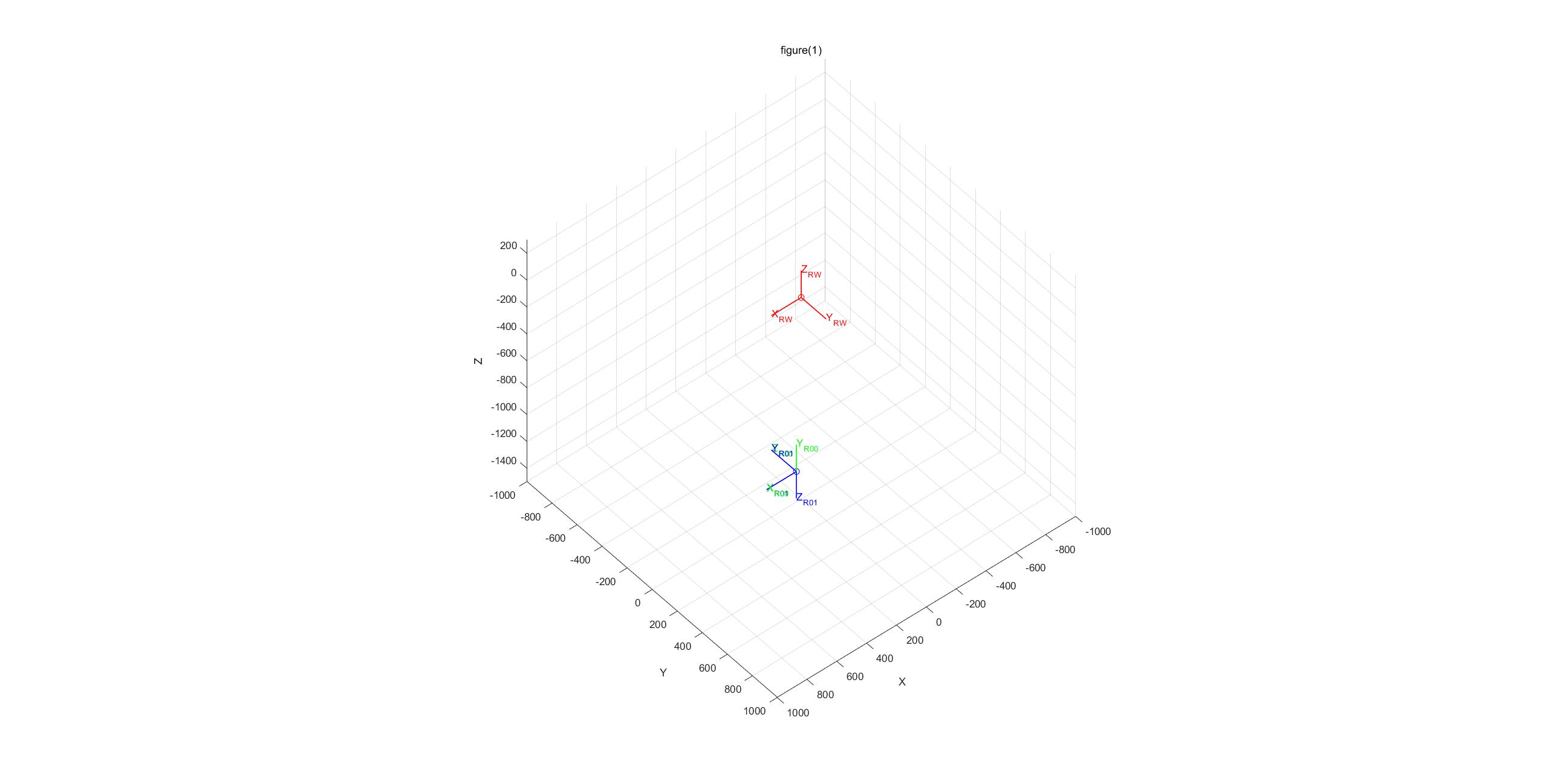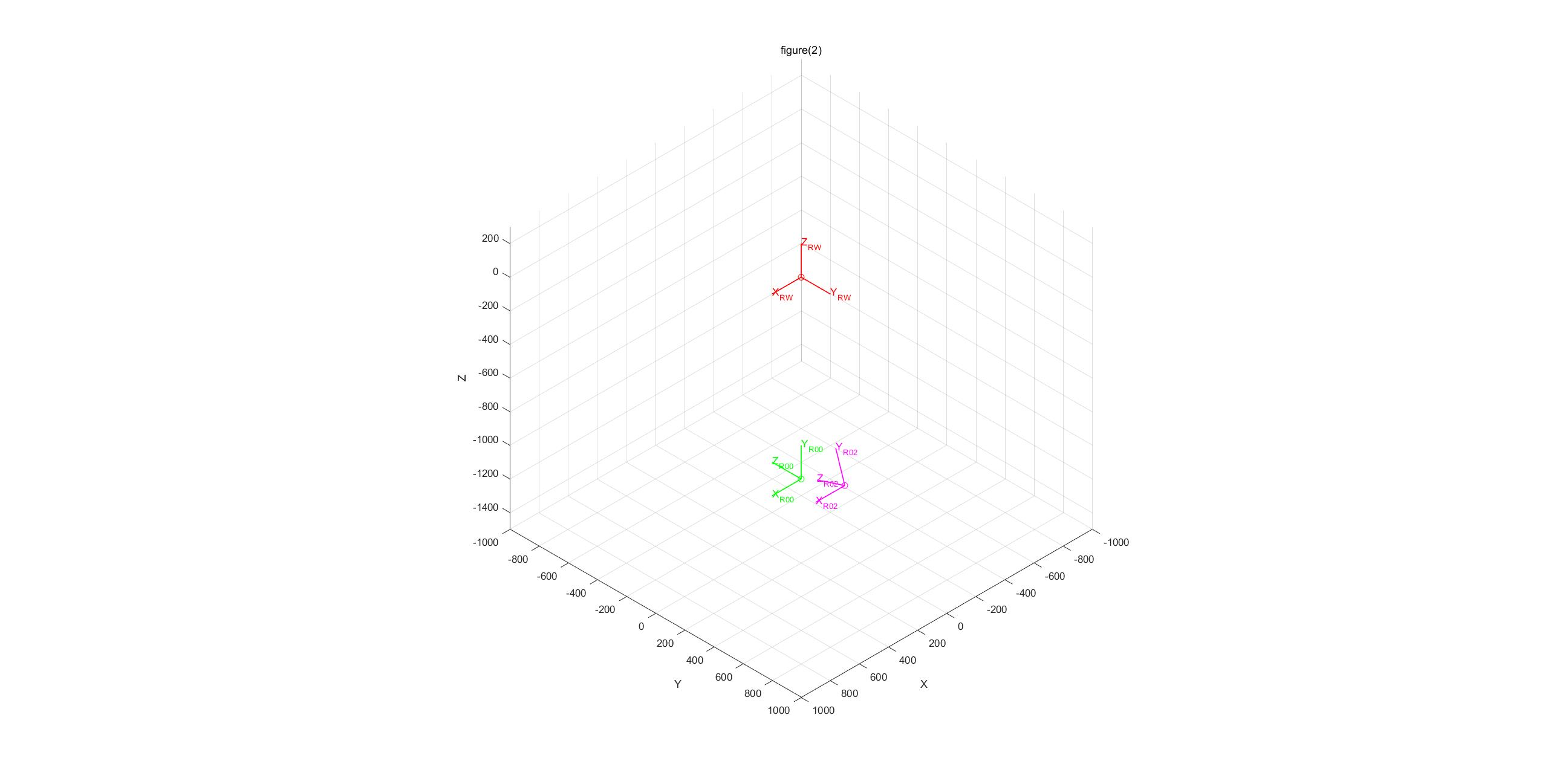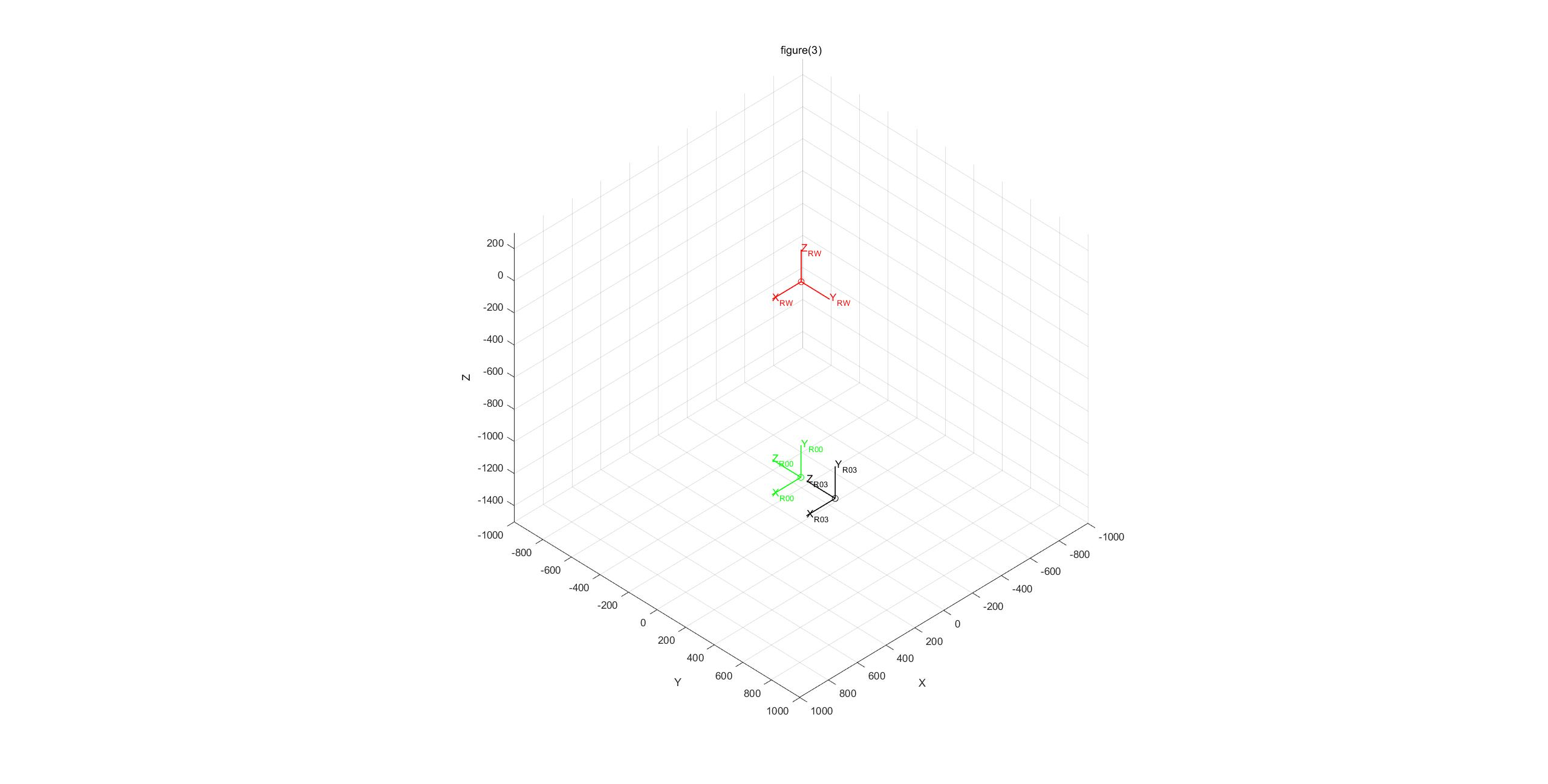​​​​​​​

展开全文• 机器人的数学基础齐次变换矩阵及其运算PPT教案.pptx
• 这组函数可以支持人们使用 Peter Corke 的 Robotics Toolbox 来管理齐次变换矩阵。 特别是，该包包含使用单个命令创建旋转和旋转平移矩阵的函数，并以度数代替弧度定义旋转角度。 它需要安装 Robotics Toolbox。matlab
• 齐次变换矩阵的三种解读2.1 坐标系表示2.2 坐标系变换2.3 点的操作算子3. 解决问题3.1 齐次变换矩阵的逆3.2 多重变换时的顺序4. 总结 1. 引言   上一篇文章我们讲到了齐次变换矩阵，表面上看我们得到了一个十分...

## 1. 引言

上一篇文章我们讲到了齐次变换矩阵，表面上看我们得到了一个十分简洁的表达式。如果你认为到这里关于齐次变换的问题已经十分明了那就大错特错了。相反，我认为这恰恰是混乱的开始。

举个例子，首先一个坐标系{A}绕其x轴旋转 α \alpha 得到坐标系{B}，根据前面讲过的知识你很容易明白坐标系{A}和{B}之间的关系为 T B A = r o t x ( α ) T_{B}^{A}=rot_{x}\left ( \alpha \right ) 。可是如果坐标系{B}再绕自身的y轴旋转 β \beta 得到坐标系{C}，那么坐标系{A}和坐标系{C}之间是什么关系呢？还有如果坐标系{B}绕坐标系{A}的y轴旋转 β \beta 得到坐标系{D}，那么坐标系{A}和坐标系{D}之间是什么关系呢？

你可能没有答案。课堂上这个问题也总是被匆匆略过，而我认为这里才是应该花大量的精力去讲解的地方。只告诉我当绕原坐标系旋转时变换矩阵要倒着乘，当绕新坐标系旋转时变换矩阵要正着乘我是无法接受的。

例子中的问题其实涉及到了齐次变换矩阵的几种解读，从这几种解读方式出发，我们很容易理解矩阵相乘的顺序到底是怎样的以及为什么是这样的。

## 2. 齐次变换矩阵的三种解读

斯坦福大学公开课—机器人学，这门课对这三种解读给出了很恰当的总结：坐标系表示（coordinate representation）、坐标系变换（coordinate transformation）、点的操作算子（point operator）。接下来我们分别对它们进行解释。

### 2.1 坐标系表示

所谓坐标系表示指的是，齐次变换矩阵可以用来表示一个坐标系。我们来看如下图两个坐标系{A}和{B}。通过上一篇文章机器人正运动学（5）—— 齐次变换矩阵的介绍我们知道坐标系{A}和坐标系{B}之间的关系为：A T B = [ A R B p B o r g A 0 1 ] ^{A}\textrm{T}_{B}=\begin{bmatrix} ^{A}\textrm{R}_{B} & p_{Borg}^{A}\\ 0 & 1 \end{bmatrix}

在文章机器人正运动学（4）—— 理解变换矩阵中我们介绍了旋转矩阵 A R B ^{A}\textrm{R}_{B} （{A}和{B}的姿态关系）代表的是坐标系{B}三个轴的单位矢量在坐标系{A}中的投影（即在坐标系{A}中坐标系{B}的三轴单位矢量的坐标），在文章机器人正运动学（5）—— 齐次变换矩阵中我们也看到 p B o r g A p_{Borg}^{A} （{A}和{B}的位置关系）代表的是坐标系{B}的原点在坐标系{A}中的坐标。

我们知道，要想唯一确定一个坐标系需要两个要素，一个是原点，一个是轴矢量。这两个要素在上面的齐次变换矩阵中已经全部包含了，因此我们说齐次变换矩阵可以用来表示一个坐标系，而 A T B ^{A}\textrm{T}_{B} 就是坐标系{B}在坐标系{A}中的表示

### 2.2 坐标系变换

所谓坐标系变换指的是，齐次变换矩阵可以用来描述一个坐标系经过怎样的平移和旋转能够变换到另一个坐标系。举个例子，两个坐标系{A}和{B}之间的齐次变换矩阵可以表示为： A T B = [ r o t x ( π / 4 ) t r a n s ( 1 , 2 , 3 ) 0 1 ] ^{A}\textrm{T}_{B}=\begin{bmatrix} rot_{x}(\pi /4) & trans(1,2,3)\\ 0 & 1 \end{bmatrix}

其中 r o t x ( π / 4 ) rot_{x}(\pi /4) 代表绕x轴旋转 π / 4 \pi/4 对应的旋转矩阵， t r a n s ( 1 , 2 , 3 ) trans(1,2,3) 代表在 x , y , z x, y,z 方向上平移1,2,3。这个时候我们就可以通过对坐标系{A}进行一些平移和旋转最终与坐标系{B}重合。这个操作过程对应于下面这张图。我们把这个操作过程分为两个步骤。

第一步将坐标系{A}沿着橘色的向量平移至与坐标系{C}重合，我们很容易知道这个平移量是trans(1,2,3)；

第二步将坐标系{C}绕它的x轴旋转至与坐标系{B}重合，同样容易知道这个旋转量是 r o t x ( π / 4 ) rot_{x}(\pi /4) 。因此我们说齐次变换矩阵可以用来描述坐标系的变换关系，而 A T B ^{A}\textrm{T}_{B} 就是坐标系{A}经过平移和旋转可以得到坐标系{B}。我们可以形象地描述（不一定恰当）这个变换为： { B } = { A } ⋆ A T B \{B\}=\{A\} \star ^{A}\textrm{T}_{B}

### 2.3 点的操作算子

所谓点的操作算子指的是，齐次变换矩阵可以用来对同一个坐标系内的点进行平移和旋转操作。注意这种理解和前面两种大不一样。前两种都是在描述两个坐标系之间的关系。在讨论齐次变换矩阵对点的操作时我们的讨论仅限于一个坐标系

举个例子在坐标系{A}中有一个点 P 0 P_{0} ，我们希望这个点绕坐标系{A}的x轴旋转 π / 4 \pi/4 得到一个新的点 P 1 P_{1} 。这个时候应该怎么做呢？很简单，我们写出如下的变换矩阵： R = r o t x ( π / 4 ) = [ 1 0 0 0 c o s ( π / 4 ) − s i n ( π / 4 ) 0 s i n ( π / 4 ) c o s ( π / 4 ) ] R=rot_{x}(\pi/4)=\begin{bmatrix} 1 & 0 & 0 \\ 0 & cos(\pi/4) & -sin(\pi/4) \\ 0 & sin(\pi/4) & cos(\pi/4) \end{bmatrix}

用这个变换矩阵去乘点 P 0 P_{0} 就可以得到点 P 1 P_{1} 啦。

如果你觉得理解这个有点困难，可以倒回到第一种理解来解释对点的操作问题。这个时候我们假设有一个坐标系{B}开始时与坐标系{A}完全重合，而点 P 0 P_{0} 固定在坐标系{B}中，这样在把点 P 0 P_{0} 绕坐标系{A}的x轴旋转 π / 4 \pi/4 时，坐标系{B}同样是绕坐标系{A}的x轴旋转 π / 4 \pi/4 。此时两个坐标系之间的变换矩阵即为 r o t x ( π / 4 ) rot_{x}(\pi /4) 。由于点 P 0 P_{0} 固定在坐标系{B}中，因此其在坐标系{B}中的坐标不变，因此经过旋转之后点 P 0 P_{0} 在坐标系{A}中的坐标即为 r o t x ( π / 4 ) ⋅ P 0 rot_{x}(\pi /4)\cdot P_{0} 。我们可以看出这个点就是前面提到的在坐标系{A}中的 P 1 P_{1} 点。

光用文字来描述似乎有些凌乱，下面我们用图把上面一段话再重新捋一遍，首先我们假设空间中一点 P 0 P_{0} 在坐标系{A}中的坐标为 P 0 ( y 0 , z 0 ) P_{0}(y_{0},z_{0}) ，为了便于看图，坐标系我们只画出 y ， z y，z 两个轴，所有点的x坐标均为0。与 P 0 P_{0} 固连的坐标系称为{B}。坐标系{B}最开始与坐标系{A}重合，如下图所示就是问题的初始状态。接下来开始旋转操作。我们是希望点 P 0 P_{0} 沿坐标系{A}的x轴旋转 π / 4 \pi/4 ，注意因为坐标系{B}和点 P 0 P_{0} 是固连在一起的，所以坐标系{B}也会绕着坐标系{A}的x轴旋转 π / 4 \pi/4 ，经过旋转之后就得到如下的图的状态。点 P 1 P_{1} 就是点 P 0 P_{0} 旋转之后对应的点。这里有一点需要特别注意，由于坐标系{B}与点 P 0 P_{0} 固连，那么经过旋转后得到的 P 1 P_{1} 点在坐标系{B}中的坐标数值实际上没有发生任何变化（和最开始的时候点 P 0 P_{0} 在坐标系{A}中的坐标值是一样的），请仔细体会这句话下面我们把变换前后的两种状态合并起来得到下面的图。至此我们可以得出这样一个结论：点 P 0 P_{0} 在坐标系{A}中的坐标值与点 P 1 P_{1} 在{B}中的坐标值是一致的。那么如何求解点 P 1 P_{1} 在坐标系{A}中的坐标值呢？仔细思考一下是不是这样一种表达： P 1 A = R B A ⋅ P 1 B P_{1}^{A}=R_{B}^{A}\cdot P_{1}^{B}

解释一下这个公式， P 1 A P_{1}^{A} 代表点 P 1 P_{1} 在坐标系{A}下的坐标。 P 1 B P_{1}^{B} 代表 P 1 P_{1} 在坐标系{B}下的坐标。还记得我们之前说的吗， P 1 P_{1} 在坐标系{B}下的坐标数值上与 P 0 P_{0} 在坐标系{A}中的坐标数值一致！以上公式可以改写为 P 1 A = R B A ⋅ P 0 A P_{1}^{A}=R_{B}^{A}\cdot P_{0}^{A}

至此我们看到 R B A R_{B}^{A} 实现了将点 P 0 P_{0} 旋转到 P 1 P_{1} 点。你也可以清晰的看到上一个公式点的上标都是A，和我们之前说的同一个空间点在不同坐标系下的表达还是有区别的。

## 3. 解决问题

### 3.1 齐次变换矩阵的逆

上一篇文章机器人正运动学（5）—— 齐次变换矩阵中我们遗留了一个问题就是齐次变换矩阵的逆。通常而言求任意矩阵的逆是比较困难的。但是由于齐次变换矩阵的良好性质以及很强的几何特征，我们很容易直接写出它的逆。

已知如下的齐次变换矩阵： A T B = [ A R B p B o r g A 0 1 ] ^{A}\textrm{T}_{B}=\begin{bmatrix} ^{A}\textrm{R}_{B} & p_{Borg}^{A}\\ 0 & 1 \end{bmatrix}

首先看旋转部分，在文章机器人正运动学（4）—— 理解变换矩阵中我们提到过 A R B ^{A}\textrm{R}_{B} 的列代表坐标系{B}的三轴矢量在坐标系{A}中的投影，行代表坐标系{A}的三轴矢量在坐标系{B}中的投影，因此， A R B ^{A}\textrm{R}_{B} 的转置就是其逆。

其次来看平移部分， p B o r g A p_{Borg}^{A} 代表坐标系{B}的原点在坐标系{A}中的坐标，而我们需要的是坐标系{A}的原点在坐标系{B}中的坐标，即 p A o r g B p_{Aorg}^{B} ，从几何的角度看，这两个向量恰好是相反向量，因此我们首先把向量 p B o r g A p_{Borg}^{A} 取反得到 − p B o r g A -p_{Borg}^{A} 。到这一步还没有完，因为目前为止这个向量是在坐标系{A}中描述的，因此我们要将它变换到坐标系{B}中的描述，即为 − A R B T ⋅ p B o r g A -^{A}\textrm{R}_{B}^{T}\cdot p_{Borg}^{A} ，其中 A R B T ^{A}\textrm{R}_{B}^{T} 代表 A R B ^{A}\textrm{R}_{B} 的转置（其实也就是 A R B ^{A}\textrm{R}_{B} 的逆）。

至此我们得到了坐标系{A}相对于坐标系{B}的姿态 A R B T ^{A}\textrm{R}_{B}^{T} ，以及坐标系{B}中描述的坐标系{A}原点坐标 p A o r g B = − A R B T ⋅ p B o r g A p_{Aorg}^{B}=-^{A}\textrm{R}_{B}^{T}\cdot p_{Borg}^{A} 。因此变换矩阵的逆即为： A T B − 1 = [ A R B T − A R B T ⋅ p B o r g A 0 1 ] = B T A = [ B R A − B R A ⋅ p B o r g A 0 1 ] ^{A}\textrm{T}_{B}^{-1}=\begin{bmatrix} ^{A}\textrm{R}_{B}^{T} & -^{A}\textrm{R}_{B}^{T}\cdot p_{Borg}^{A}\\ 0 & 1 \end{bmatrix}=^{B}\textrm{T}_{A}=\begin{bmatrix} ^{B}\textrm{R}_{A} & -^{B}\textrm{R}_{A}\cdot p_{Borg}^{A}\\ 0 & 1 \end{bmatrix}

### 3.2 多重变换时的顺序

在此我们解决文章开头提出的多重变换时如何排列变换矩阵。其实就是两种情况，第一种情况是所有的变换都相对于最开始的坐标系；第二种情况是所有变换都相对于新得到的坐标系。我们分情况讨论。

首先来看第一种情况，坐标系{A}绕其x轴旋转 α \alpha 得到坐标系{B}，之后坐标系{B}又绕坐标系{A}的y轴旋转 β \beta 得到坐标系{C}。此时我们用对齐次变换矩阵的第三种理解来求得坐标系{C}和坐标系{A}之间的关系。我们假设在坐标系{A}中有一个点 P 0 P_{0} ，在第一次变换时我们假设有一个坐标系{T1}与坐标系{A}重合，同时点 P 0 P_{0} 固定在坐标系{T1}中，经过第一次变换后得到在{A}中新的点 P 1 P_{1} 的坐标为 r o t x ( α ) ⋅ P 0 rot_{x}(\alpha )\cdot P_{0} （注意这是 P 1 P_{1} 点在坐标系{A}中的坐标）。在第二次变换时我们假设又有一个坐标系{T2}与坐标系{A}重合，同时点 P 1 P_{1} 又固定在坐标系{T2}中，经过第二次变换后得到在{A}中新的点 P 2 P_{2} 的坐标为 r o t y ( β ) ⋅ P 1 = r o t y ( β ) ⋅ r o t x ( α ) ⋅ P 0 rot_{y}(\beta )\cdot P_{1}=rot_{y}(\beta )\cdot rot_{x}(\alpha )\cdot P_{0} ，因此我们可以最终确定坐标系{A}和{C}之间的变换关系为： A R C = r o t y ( β ) ⋅ r o t x ( α ) ^{A}\textrm{R}_{C}=rot_{y}(\beta )\cdot rot_{x}(\alpha )

再来看第二种情况，坐标系{A}绕其x轴旋转 α \alpha 得到坐标系{B}，之后坐标系{B}又绕其y轴旋转 β \beta 得到坐标系{C}（注意这里是绕坐标系{B}的y轴）。此时我们用齐次变换矩阵的第二种理解来求坐标系{C}和坐标系{A}之间的关系。设空间中有一个点 P P ，它在坐标系{C}中的坐标为 P C P_{C} ，我们首先看坐标系{B}和坐标系{C}之间的关系，由于{B}绕自身的y轴旋转 β \beta 就可以得到{C}，因此，同一个空间点 P P 在坐标系{B}中的坐标为 P B = r o t y ( β ) ⋅ P C P_{B}=rot_{y}(\beta )\cdot P_{C} 。我们又知道坐标系{A}绕其x轴旋转 α \alpha 得到坐标系{B}，因此，同一个空间点P在坐标系{A}中的坐标为 P A = r o t x ( α ) ⋅ P B = r o t x ( α ) ⋅ r o t y ( β ) ⋅ P C P_{A}=rot_{x}(\alpha )\cdot P_{B}=rot_{x}(\alpha )\cdot rot_{y}(\beta )\cdot P_{C} ，因此我们可以最终确定坐标系{A}和{C}之间的变换关系为： A R C = r o t x ( α ) ⋅ r o t y ( β ) ^{A}\textrm{R}_{C}=rot_{x}(\alpha )\cdot rot_{y}(\beta )

这也验证了文章开头提出的结论，那就是绕着原坐标系旋转就倒着乘，绕着新坐标系旋转就正着乘

## 4. 总结

这篇文章主要介绍了齐次变换矩阵的三种理解以及解决了一个比较容易造成混乱的多重变换时变换矩阵的顺序问题。由于个人能力有限，所述内容难免存在疏漏，欢迎指出，欢迎讨论。

下一篇：机器人正运动学（7）—— 连杆坐标系与DH参数

展开全文• 这篇文章介绍了我们可以从三个不同的角度理解齐次变换矩阵。利用这三种解读可以帮助我们轻松地去理解一些齐次变换过程，特别是齐次变换矩阵的乘法顺序问题。

目录

1. 引言

2. 齐次变换矩阵的三种解读

2.1 坐标系表示

2.2 坐标系变换

2.3 点的操作算子

3. 解决问题

3.1 齐次变换矩阵的逆

3.2 多重变换时的顺序

4. 总结

# 1. 引言

上一篇文章我们讲到了齐次变换矩阵，表面上看我们得到了一个十分简洁的表达式。如果你认为到这里关于齐次变换的问题已经十分明了那就大错特错了。相反，我认为这恰恰是混乱的开始。

举个例子，首先一个坐标系{A}绕其轴旋转得到坐标系{B}，根据前面讲过的知识你很容易明白坐标系{A}和{B}之间的关系为。可是如果坐标系{B}再绕自身的轴旋转得到坐标系{C}，那么坐标系{A}和坐标系{C}之间是什么关系呢？还有如果坐标系{B}绕坐标系{A}的轴旋转得到坐标系{D}，那么坐标系{A}和坐标系{D}之间是什么关系呢？

你可能没有答案。课堂上这个问题也总是被匆匆略过，而我认为这里才是应该花大量的精力去讲解的地方。只告诉我当绕原坐标系旋转时变换矩阵要倒着乘，当绕新坐标系旋转时变换矩阵要正着乘我是无法接受的。

例子中的问题其实涉及到了齐次变换矩阵的几种解读，从这几种解读方式出发，我们很容易理解矩阵相乘的顺序到底是怎样的以及为什么是这样的。

# 2. 齐次变换矩阵的三种解读

斯坦福大学公开课---机器人学，这门课对这三种解读给出了很恰当的总结：坐标系表示（coordinate representation）、坐标系变换（coordinate transformation）、点的操作算子（point operator）。接下来我们分别对它们进行解释。

## 2.1 坐标系表示

所谓坐标系表示指的是，齐次变换矩阵可以用来表示一个坐标系。我们来看如下图两个坐标系{A}和{B}。通过上一篇文章机器人正运动学---齐次变换矩阵的介绍我们知道坐标系{A}和坐标系{B}之间的关系为：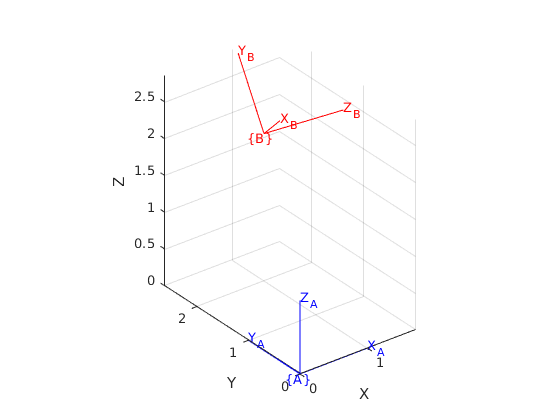在文章机器人正运动学---理解变换矩阵中我们介绍了旋转矩阵（{A}和{B}的姿态关系）代表的是坐标系{B}三个轴的单位矢量在坐标系{A}中的投影（即在坐标系{A}中坐标系{B}的三轴单位矢量的坐标），在文章机器人正运动学---齐次变换矩阵中我们也看到（{A}和{B}的位置关系）代表的是坐标系{B}的原点在坐标系{A}中的坐标。

我们知道，要想唯一确定一个坐标系需要两个要素，一个是原点，一个是轴矢量。这两个要素在上面的齐次变换矩阵中已经全部包含了，因此我们说齐次变换矩阵可以用来表示一个坐标系，而就是坐标系{B}在坐标系{A}中的表示

## 2.2 坐标系变换

所谓坐标系变换指的是，齐次变换矩阵可以用来描述一个坐标系经过怎样的平移和旋转能够变换到另一个坐标系。举个例子，两个坐标系{A}和{B}之间的齐次变换矩阵可以表示为：

其中代表绕轴旋转对应的旋转矩阵，，代表在方向上平移1,2,3。这个时候我们就可以通过对坐标系{A}进行一些平移和旋转最终与坐标系{B}重合。这个操作过程对应于下面这张图。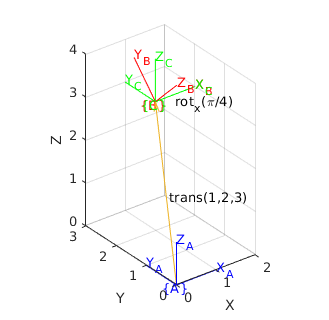我们把这个操作过程分为两个步骤。

第一步将坐标系{A}沿着橘色的向量平移至与坐标系{C}重合，我们很容易知道这个平移量是

第二步将坐标系{C}绕它的轴旋转至与坐标系{B}重合，同样容易知道这个旋转量是。因此我们说齐次变换矩阵可以用来描述坐标系的变换关系，而就是坐标系{A}经过平移和旋转可以得到坐标系{B}。我们可以形象地描述（不一定恰当）这个变换为：

## 2.3 点的操作算子

所谓点的操作算子指的是，齐次变换矩阵可以用来对同一个坐标系内的点进行平移和旋转操作。注意这种理解和前面两种大不一样。前两种都是在描述两个坐标系之间的关系。在讨论齐次变换矩阵对点的操作时我们的讨论仅限于一个坐标系。

举个例子在坐标系{A}中有一个点，我们希望这个点绕坐标系{A}的轴旋转得到一个新的点。这个时候应该怎么做呢？很简单，我们写出如下的变换矩阵：

用这个变换矩阵去乘点就可以得到点啦。

如果你觉得理解这个有点困难，可以倒回到第一种理解来解释对点的操作问题。这个时候我们假设有一个坐标系{B}开始时与坐标系{A}完全重合，而点又固定在坐标系{B}中，这样在把点绕坐标系{A}的轴旋转时，坐标系{B}同样是绕坐标系{A}的轴旋转。此时两个坐标系之间的变换矩阵即为。由于点固定在坐标系{B}中，因此其在坐标系{B}中的坐标不变，因此经过旋转之后点在坐标系{A}中的坐标即为。我们可以看出这个点就是前面提到的在坐标系{A}中的点。

光用文字来描述似乎有些凌乱，下面我们用图把上面一段话再重新捋一遍，首先我们假设空间中一点在坐标系{A}中的坐标为，为了便于看图，坐标系我们只画出y，z两个轴，所有点的x坐标均为0。与点固连的坐标系称为{B}。坐标系{B}最开始与坐标系{A}重合，如下图所示就是问题的初始状态。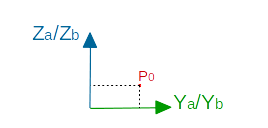接下来开始旋转操作。我们是希望点沿坐标系{A}的轴旋转，注意因为坐标系{B}和点是固连在一起的，所以坐标系{B}也会绕着坐标系{A}的轴旋转，经过旋转之后就得到如下的图的状态。点就是点旋转之后对应的点。这里有一点需要特别注意，由于坐标系{B}与点固连，那么经过旋转后得到的点在坐标系{B}中的坐标数值实际上没有发生任何变化（和最开始的时候点在坐标系{A}中的坐标值是一样的），请仔细体会这句话。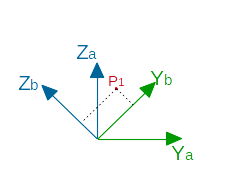下面我们把变换前后的两种状态合并起来得到下面的图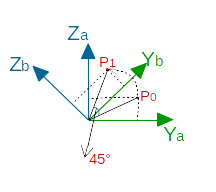至此我们可以得出这样一个结论，点在坐标系{A}中的坐标值与点在{B}中的坐标值是一致的。那么如何求解点在坐标系{A}中的坐标值呢？仔细思考一下是不是这样一种表达：

解释一下这个公式，代表点在坐标系{A}下的坐标。代表在坐标系{B}下的坐标。还记得我们之前说的吗，在坐标系{B}下的坐标数值上与在坐标系{A}中的坐标数值一致！以上公式可以改写为

至此我们看到实现了将点旋转到点。你也可以清晰的看到上一个公式点的上标都是A，和我们之前说的同一个空间点在不同坐标系下的表达还是有区别的。

# 3. 解决问题

## 3.1 齐次变换矩阵的逆

上一篇文章机器人正运动学---齐次变换矩阵中我们遗留了一个问题就是齐次变换矩阵的逆。通常而言求任意矩阵的逆是比较困难的。但是由于齐次变换矩阵的良好性质以及很强的几何特征，我们很容易直接写出它的逆。

已知如下的齐次变换矩阵：

首先看旋转部分，在文章机器人正运动学---理解变换矩阵中我们提到过的列代表坐标系{B}的三轴矢量在坐标系{A}中的投影，行代表坐标系{A}的三轴矢量在坐标系{B}中的投影，因此，的转置就是其逆。

其次来看平移部分，代表坐标系{B}的原点在坐标系{A}中的坐标，而我们需要的是坐标系{A}的原点在坐标系{B}中的坐标，即，从几何的角度看，这两个向量恰好是相反向量，因此我们首先把向量取反得到。到这一步还没有完，因为目前为止这个向量是在坐标系{A}中描述的，因此我们要将它变换到坐标系{B}中的描述，即为，其中代表的转置。

至此我们得到了坐标系{A}相对于坐标系{B}的姿态，以及坐标系{B}中描述的坐标系{A}原点坐标。因此变换矩阵的逆即为：

## 3.2 多重变换时的顺序

在此我们解决文章开头提出的多重变换时如何排列变换矩阵。其实就是两种情况，第一种情况是所有的变换都相对于最开始的坐标系；第二种情况是所有变换都相对于新得到的坐标系。我们分情况讨论。

首先来看第一种情况，坐标系{A}绕其轴旋转得到坐标系{B}，之后坐标系{B}又绕坐标系{A}的轴旋转得到坐标系{C}。此时我们用对齐次变换矩阵的第三种理解来求得坐标系{C}和坐标系{A}之间的关系。我们假设在坐标系{A}中有一个点，在第一次变换时我们假设有一个坐标系{T1}与坐标系{A}重合，同时点固定在坐标系{T1}中，经过第一次变换后得到在{A}中新的点的坐标为（注意这是点在坐标系{A}中的坐标）。在第二次变换时我们假设又有一个坐标系{T2}与坐标系{A}重合，同时点又固定在坐标系{T2}中，经过第二次变换后得到在{A}中新的点的坐标为，因此我们可以最终确定坐标系{A}和{C}之间的变换关系为：

再来看第二种情况，坐标系{A}绕其轴旋转得到坐标系{B}，之后坐标系{B}又绕其轴旋转（注意这里是绕坐标系{B}的轴）。此时我们用齐次变换矩阵的第二种理解来求坐标系{C}和坐标系{A}之间的关系。设空间中有一个点，它在坐标系{C}中的坐标为，我们首先看坐标系{B}和坐标系{C}之间的关系，由于{B}绕自身的轴旋转就可以得到{C}，因此，同一个空间点在坐标系{B}中的坐标为。我们又知道坐标系{A}绕其轴旋转得到坐标系{B}，因此，同一个空间点在坐标系{A}中的坐标为，因此我们可以最终确定坐标系{A}和{C}之间的变换关系为：

这也验证了文章开头提出的结论，那就是绕着原坐标系旋转就倒着乘，绕着新坐标系旋转就正着乘。

# 4. 总结

这篇文章主要介绍了齐次变换矩阵的三种理解以及解决了一个比较容易造成混乱的多重变换时变换矩阵的顺序问题。由于个人能力有限，所述内容难免存在疏漏，欢迎指出，欢迎讨论。

下一篇：7. 机器人正运动学---连杆坐标系与DH参数

展开全文正运动学
•机器人学基础
• ## 齐次变换矩阵

千次阅读 2020-01-11 11:48:06
机器人中齐次变换阵（4*4...齐次变换矩阵可以用作： （1）描述坐标系，表示坐标系B在坐标系A中的描述。左上角3*3的旋转矩阵。 （2）用作变换映射：将B中的矢量在A中表示。 （3）变换算子：A中的矢量平移和旋转。 ...
• 齐次坐标系变换2.1 坐标系之间的位姿关系2.2 齐次变换矩阵2.3 齐次变换矩阵的逆4. 总结 1. 引言   前面的文章中我们分别讨论了坐标系及其平移，旋转两种变换。但是到目前为止我们一直都在分开讨论平移和旋转，而...
• 已知坐标系 AAA 相对于坐标系 BBB 的齐次变换矩阵为 TABT_{A}^{B}TAB​，有时在求解问题时需要依据 TABT_{A}^{B}TAB​ 求取坐标系 BBB 相对于坐标系 AAA 的齐次变换矩阵 TBAT_{B}^{A}TBA​ ，这个问题可以简单的描述...
• ## 5. 机器人正运动学---齐次变换矩阵

万次阅读 多人点赞 2019-12-31 23:04:14
这篇文章主要介绍了齐次变换矩阵的由来以及它的逆。机器人学 机器人正运动学
• 在机器人及自动驾驶中，经常用其次变换矩阵将旋转和平移进行统一。 前面的文章也介绍过其次变换矩阵，本文算是一个总结。 1. SE(3) 将旋转矩阵和平移向量写在同一个矩阵中，形成的4×44\times44×4矩阵，称为special...自动驾驶
• 对于齐次变换矩阵之间的相乘，我们以三个坐标系之间的变换关系为例： 是{C}在{A}中的描述，是{B}在{A}中的描述，是{C}在{B}中的描述。 对于坐标系之间的运动过程，我们可以做如下理解： （1） 第一步：{C}相...算法
• 6. 机器人正运动学---齐次变换矩阵的三种解读_hitgavin的博客-CSDN博客_齐次变换矩阵 相机标定坐标系转换表示 SLAM入门之视觉里程计(6)：相机标定 张正友经典标定法详解 - Brook_icv - 博客园
• 齐次变换矩阵 nx ny nz 为运动坐标系中三个轴对应 得单位方向向量在参考坐标系中投影，Px,Py,Pz.为坐标原点在参考系中得位置。单位方向向量模长为1，两两正交 齐次变换矩阵T 纯平移 绕轴纯旋转 相当于把一个在R...
• 齐次变换矩阵的意义+坐标变换公式+坐标变换动画展示css3
• tf是很常用的消息类型，但有时候我们更希望直到他的四乘四的齐次变换矩阵。 源代码 这个代码是辅助velo2cam_calibration用的，实现两个tf的接收，得到相机到雷达的坐标系转换关系。 大家完全可以根据自己需求修改...自动驾驶 c++
• 齐次变换矩阵 坐标系{B}原点相对于坐标系{A}原点的偏移可以用矢量APBORG表示，同时{B}相对于{A}的旋转可以用ABR描述。已知BP，可得到： 由上式变换可得： 因此由BP到AP的变换可描述为： ABT就被称为齐次变化矩阵...
• 相对于参考坐标系是左乘，相对于当前坐标系是右成，因此图中的就可以理解为，i...值得注意的是，在整个某个p在zi的坐标系下的位姿转换到zi-1上面的时候是左乘变换矩阵T,大概的意思就是将zi-1坐标系当做参考坐标。 ...
• 旋转矩阵的初衷就是人们希望给定一个向量x，然后我对它旋转，能直接通过矩阵乘法的形式得到旋转后的向量坐标。也就是说y=Ax。这个方便计算机计算，因此旋转矩阵常用于编程。旋转矩阵是一个正交矩阵:自动驾驶
• 欧拉公式求长期率的matlab代码齐次变换矩阵和四元数 Transformations是一个Python库，用于计算4x4矩阵，以平移，旋转，反射，缩放，剪切，投影，正交化和叠加3D齐次坐标数组，以及在旋转矩阵，欧拉角和四元数之间...
• 对于齐次位姿变换（RT矩阵），有的时候手动计算公式参数太多比较麻烦，因此利用matlab参量syms，可以方便一些，可以用于计算机器人正运动学位姿矩阵的参数表示，简单的小程序，写机器人学作业的时候可能有点儿用，...
• 旋转矩阵的初衷就是人们希望给定一个向量x，然后我对它旋转，能直接通过矩阵乘法的形式得到旋转后的向量坐标。也就是说y=Ax。这个方便计算机计算，因此旋转矩阵常用于编程。既然有了旋转矩阵那么为何还要欧拉角呢？...旋转矩阵 旋转向量
• 这个很多人都会直接说：“就是不可逆啊，你拿两个矩阵乘以下，在换个顺序乘以下，它们的结果就是不一样的。”是啊，但是为什么它们的结果是不一样的呢？小虎曾经在机器人学课堂上问过老师，老师含糊其词，想拿上面那...机器人学
• 齐次变换矩阵及其运算 由于各种原因，变换矩阵应该写成方型形式，33或者44即可。 为保证所表示的矩阵为方阵，如果在同一矩阵中既表示姿态又表示位置，那么在矩阵中加入比例因子使之成为4*4的矩阵即可。 变换可以......